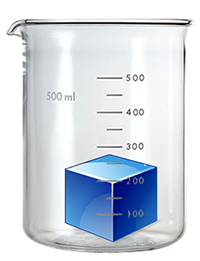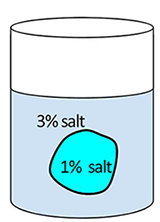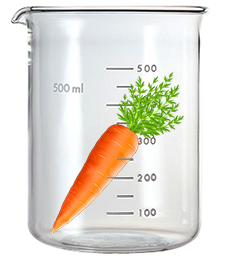Name:____________________________________________Date: _______## Investigation:  Modeling Cells in Solutions

Osmosis is the movement of water across a selectively permeable membrane, meaning that it allows some things to cross it, but not others.  The cell membrane is permeable to water, which means that water can move across the membrane.  Water moves in the direction where there is the most solute.   Solutes are just particles that have been dissolved in water, like sugar or salt, or other cellular materials.   Water will move toward the area with the greatest solute concentration.   For example, if you place a cell in a salty solution, the water in the cell will move out of the cell, causing the cell to shrink.

There are three different types of solutions:

Hypotonic =  solution has LESS solutes than the cell
Hypertonic = solution has MORE solutes than the cell
Isotonic = solution has the same amount of solute as the cell

### Pre-Lab Questions1.  Osmosis is the movement of  [ solutes /  water  ] across a membrane
2.  A cell that is 1% salt is placed in a solution that is 3% salt.  This solution is
[ hypotonic / hypertonic / isotonic ]
3.  The cell placed in this solution will [ gain / lose ] water.
4.  The cell will then [ shrink  / swell ].
5.   The cell membrane is selectively _________________________________,

Modeling Osmosis

1. Obtain 3 deco-cubes that have been soaked overnight.  These cubes are absorb water and are used as decoration in flower vases.
2. Measure each cube side in millimeters (length, width, height).  Calculate the volume of each cube and complete the data table.   →  Volume = length x width x height.
3.  Place the cubes in the following solutions:   Salt water  |   Tap Water |  Distilled Water .
4.  Let cubes sit for 24 hours and measure the volume of each the next day.

 DATA TABLE Volume (Day 1) Volume (Day 2) Cube 1 (Tap Water) Cube 2 ( Salt Water) Cube 3 (Distilled Water)

### Analysis

1.  Which solution was hypertonic relative to the cube?      How do you know?

2.  Which solution was hypotonic relative to the cube?    How do you know?

3.  How does the cube serve as a model for the cell?  (Consider properties it has that is similar to a cell.)

4.  What do you think would happen to a carrot that was soaked overnight in salt water?   Explain your reasoning.

5.  Describe what would happen to a blood cell that was placed in a HYPOTONIC solution.